# Focal Length of a Concave Mirror and a Convex Lens using U-V Method

### 1 Introduction

The sign conventions are important in geometrical optics. All distances are measured from the pole. Distances measured in the direction of the incident ray are positive and the distances measured in the direction opposite to that of the incident rays are negative. Distances measured above the principal axis are positive and that measured below the principal axis are negative.

The object distance u, image distance v and the focal length f for a spherical mirror are related by the mirror formula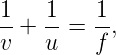and for the spherical lens are related by the lens formula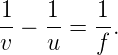The u-v method to find focal length of a given concave mirror or convex lens consists of following steps

1. For an appropriate object distance u, find the image distance v. Measure u and v.
2. Repeat above step at few more object distances. Get the focal length from all measurements by (a) taking average of calculated f or (b) from u versus v graph or (c) fromversusgraph.

#### 1.1 Concave Mirror

Look at the ray diagram shown in the figure. The distances are measured from the pole P. The incident ray (from the object to the mirror) is from the left to the right. Thus, u, v and f are all negative (by sign convention).

The u versus v graph is shown in the figure. The important points in this figure are:

1. The graph is hyperbola with asymptotes at u = f and v = f i.e., for the object placed at F the image is formed at infinity and for the object placed at infinity the image is formed at F.
2. The values of u and v are equal at point C, which corresponds to u = v = 2f. This point is the intersection of u-v curve and the straight line v = u. This represents centre of curvature of the mirror.

The 1∕u versus 1∕v graph is shown in the figure. The graph is a straight line with slope -1 and intercept on the 1∕v-axis is 1∕f. Note that 1∕u is zero when the object is placed at infinity. In this case, the image is formed at the focus i.e., v = f.

#### 1.2 Convex Lens

Look at the ray diagram shown in the figure. Note that u is negative, but v and f are positive (by sign convention).

The u versus v graph is shown in the figure. The important points in the figure are:

1. The graph is hyperbola with asymptotes at u = -f and v = f i.e., for the object placed at F the image is formed at infinity and for the object at infinity the image is formed at F.
2. At the point C, the values of u and v are equal in magnitude but opposite in sign i.e., v = -u = 2f. This point is the intersection of u-v curve and the straight line v = -u. If an object is placed at a distance 2f from the pole then its image is formed at a distance 2f from the pole (on the other side).

The 1∕u versus 1∕v graph is shown in the figure. The graph is a straight line with slope 1 and intercept on 1∕v-axis is 1∕f. Note that 1∕u is zero when object is placed at infinity. In this case, the image is formed at the focus i.e., v = f.

### 2 IIT JEE Solved Problems

Question. In an experiment to determine the focal length (f) of a concave mirror by the u-v method, a student places the object pin A on the principal axis at a distance x from the pole P. The student looks at the pin and its inverted image from a distance keeping his/her eye in line with PA. When the student shifts his/her eye towards left, the image appears to the right of the object pin. Then, (IIT JEE 2007)

1. x < f
2. f < x < 2f
3. x = 2f
4. x > 2f

Solution. The concave mirror forms the inverted image only if object is placed beyond the focus i.e., x > f. Also, if x = 2f then the object and the image coincides with each other and the student cannot observe parallax.

In general, the concept of parallax is memorized by the students without understanding it. Let A and B be two points in space. Place your eye at E on the line AB (see figure). If you move eye in one direction then the point A, which is near to the eye, appears to move in the opposite direction. On the other hand, the point B, which is far from the eye, appears to move in the direction of eye movement. Move the eye leftward from the point E to the point E1. The line joining the eye to the far point B moves from EB to E1B and the near point A appears to move rightward relative to this line. The line joining the eye to the near point A move from EA to E1A and the far point B appears to move rightward relative to this line. The readers are encouraged to experience this phenomenon by placing forefingers of two hands in front of their eyes and then moving the head to change eye’s position. If the points A and B coincides with each other then parallax is not observed i.e., parallax between A and B is removed.

In given case, when eye is shifted towards left, the image appears to the right of the object pin. Thus, image lies between the eye and the object pin. Hence, the object is near to the pole P in comparison to its image. This is possible when the object is placed between f and 2f i.e., f < x < 2f.

Question. A student performed the experiment for determination of focal length of a concave mirror by using u-v method using an optical bench of length 1.5m. The focal length of the mirror used is 24cm. The maximum error in the location of the image can be 0.2cm. The 5 sets of (u,v) values recorded by the student (in cm) are: (42, 56), (48, 48), (60, 40), (66, 33) and (78, 39). The data set(s) that cannot come from experiment and is (are) incorrectly recorded, is (are) (IIT JEE 2009)

1. (42, 56)
2. (48, 48)
3. (66, 33)
4. (78, 39)

Solution. Using mirror formula, 1∕v + 1∕u = 1∕f, calculate v for given u of five measurements. The (u,v) pairs with calculated values of v are (42, 56), (48, 48), (60, 40), (66, 37.7) and (78, 34.7). The absolute error in v for the last two measurements is 37.7 - 33 = 4.7cm and 39 - 34.7 = 4.3cm, which is more than maximum error in v (0.2cm).

Question. The graph between object distance u and the image distance v for a lens is as shown. The focal length of the lens is (IIT JEE 2006)

1. 5 ± 0.1
2. 5 ± 0.05
3. 0.5 ± 0.1
4. 0.5 ± 0.05

Solution. Substitute u = -10cm and v = 10cm in the lens formula,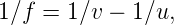to get f = 5.0cm. Differentiate above equation and simplify to get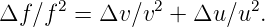Substitute u = -10cm, v = 10cm, Δu = 0.1cm, Δv = 0.1cm, and f = 5.0cm in above equation to get Δf = 0.05cm. The readers are encouraged to get the answer by differentiating f = uv∕(u - v) also.

Question. A diminished image of an object is to be obtained on a screen 1.0m from it. This can be achieved by placing (IIT JEE 1995)

1. a concave mirror of suitable focal length.
2. a convex mirror of suitable focal length.
3. a convex lens of focal length less than 0.25m.
4. a concave lens of suitable focal length.

Solution. The images formed by convex mirror and concave lens are virtual and cannot be obtained on the screen. Thus, only a concave mirror or a convex lens can be used.

Consider the case when we place a concave mirror (see figure). Let u = -x1, v = -x2, and f = -x3, where x1, x2, and x3 are positive. The magnification is given by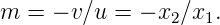For a diminished image, |m| < 1, which gives x2 < x1. The distance between the object and the image is d = x1 - x2. The mirror formula, 1∕v + 1∕u = 1∕f, givesThus, if we take x1 = 2d then we get a real image at x2 = d with magnification m = -12 by using a concave mirror of focal length f = -x3 = -2d3. However, there is a practical problem in the use of a concave mirror. The screen obstructs the rays going to the mirror.

Now, consider the case when we place a convex lens. Let u = -x1, v = x2, and f = x3, where x1, x2, and x3 are positive. The magnification is given byFor diminished image, |m| < 1, which gives x2 < x1. The distance between the object and the image is d = x1 + x2. The lens formula, 1∕v - 1∕u = 1∕f, gives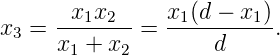The condition x2 < x1 and x1 + x2 = d gives x1 > d∕2 which sets an upper limit on the focal length fmax = x3,max = d∕4. The readers are encouraged to prove analytically or graphically that x3,max = d∕4 which occurs at x1 = d∕2.

Question. Which of the following form(s) a virtual and erect image for all positions of the object? (IIT JEE 1996)

1. Convex lens
2. Concave lens
3. Convex mirror
4. Concave mirror

Solution. The concave lens and convex mirror form virtual and erect images for all positions of the object. The readers are encouraged to verify this by their ray diagrams and/or by analysing lens and mirror formula.

Question. A converging lens is used to form an image on a screen. When the upper half of the lens is covered by an opaque screen, (IIT JEE 1986)

1. half of the image will disappear.
2. complete image will be formed.
3. intensity of the image will increase.
4. intensity of the image will decrease.

Solution. The image formed by the lens will be complete even if upper half of the lens is covered. The intensity of the image decreases because energy at each image point will be coming from lower part only. The readers are encouraged to draw ray diagram and show that image is complete.

### 3 Experiment Details

#### 3.1 Procedure

The procedure to find focal length of a convex lens by u-v method is given in NCERT book . The procedure to find focal length of a concave mirror by u-v method is given below:

1. Fix the given concave mirror on the stand. Arrange the screen on the table so that the image of the distant object is obtained on it. Measure the distance between mirror and screen using a metre scale. This distance is the approximate focal length (f) of the mirror.
2. Set the values of u ranging from 1.5f to 2.5f. Divide the range into a number of equal steps.
3. Place the mirror in front of an illuminated object. Now, fix the mirror at the distance u (which is obtained as 1.5f).
4. Place the screen on the table facing the mirror in such a way that the reflected image lies on the screen. Keeping the distance between object and mirror fixed, adjust the position of screen in order to get the clear image of the object. Remove the parallax to get accurate position of the image.
5. Measure the distance between mirror and object, as well as mirror and screen. Take these values as u and v respectively. Calculate the focal length of the given concave mirror by using the relation, f = uv∕(u+v).
6. Repeat the experiment for different values of u (up to 2.5f) and in each time, measure v and record it in the tabular column. Calculate the focal length (f) of the concave mirror each time.
7. Calculate the mean of all focal lengths to get the correct focal length of the given concave mirror.
8. The focal length of the mirror can also be measured graphically by plotting graphs between u and v, and 1∕u and 1∕v.

#### 3.2 Precautions

Some of the precautions and sources of errors are given below

1. The principal axis of the mirror should be horizontal and parallel to central line of the optical bench.
2. The object should be vertical.
3. Index correction for u and v should be applied.

#### 3.3 Data Table

The data should be recorded in the tabular format. One possible format is given below:

 S.No. u v 1∕u 1∕v f (cm) (cm) (cm-1) (cm-1) (cm)

### 4 Exercise Problems

Problem 1. An object is placed between the pole and focus of a concave mirror produces a virtual and enlarged image. Justify this using mirror formula?

Problem 2. You read a newspaper, because of the light if reflects. Then why do you not see even a faint image of yourself in the newspaper?

Problem 3. A person is standing before a concave mirror cannot see his image, unless he is beyond the centre of curvature. Why?

Problem 4. A convex lens made up of glass of refractive index 1.5 is dipped in turn in (i) medium A of refractive index 1.65 and (ii) medium B of refractive index 1.33. Explain giving reason whether it will behave as a converging lens or diverging lens in each of these two media.

Problem 5. The image distance (v) is plotted against the object distance (u) for a concave mirror of focal length f. Which of the graphs shown below represents the variation v versus u as u is varied from zero to infinity?

Problem 6. A convex lens is placed between an object and a screen which are a fixed distance apart. For one position of the lens the magnification of the image obtained on the screen is m1. When the lens is moved by a distance d, the magnification of the image obtained on the same screen is m2. The focal length of the lens is (m1 > m2)

1. d∕(m1 - m2)
2. d∕(m1 + m2)
3. dm1∕m2
4. dm2∕m1

Problem 7. The distance between the object and the real image formed by a convex lens is d. If the magnification is m, the focal length of the lens is

1. md(m + 1)2
2. md(m + 1)
3. md(m - 1)2
4. md(m - 1)

Problem 8. When an object is at distances x and y from a lens, a real image and a virtual image is formed respectively having same magnification. The focal length of the lens is

1. (x + y)2
2. x - y
3.4. x + y

Problem 9. A convex lens forms a real image three times larger than the object on a screen. Object and screen are moved until the image become twice the size of object. If the shift of the object is 6 cm. The shift of the screen is

1. 36 cm
2. 72 cm
3. 18 cm
4. 9 cm

Problem 10. If the distances of an object and its virtual image from the focus of a convex lens of focal length f are 1 cm each, then f is

1. 4 cm
2. (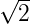+ 1) cm
3. 2cm
4. (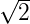+ 2) cm

Problem 11. A concave mirror has a focal length 20 cm. The distance between the two positions of the object for which the image size is double of the object size is

1. 20 cm
2. 40 cm
3. 30 cm
4. 60 cm

Problem 12. Optic axis of a thin equiconvex lens is the x-axis. The coordinates of a point object and its image are (-40 cm, 1 cm) and (50 cm, -2 cm), respectively. Lens is located at

1. x = +20 cm
2. x = -30 cm
3. x = -10 cm
4. x = 0 cm

Problem 13. A point object is placed on the optic axis of a convex lens of focal length f at a distance of 2f to the left of it. The diameter of the lens is d. An eye is placed at a distance of 3f to the right of the lens and a distance h below the optic axis. The maximum value of h to see the image is

1. d
2. d∕2
3. d∕3
4. d∕4

Problem 14. For a concave mirror

1. virtual image is always larger in size
2. real image is always smaller in size
3. real image is always larger in size
4. real image may be larger or smaller in size

Problem 15. If a man’s face is 20 cm in front of concave shaving mirror creating an upright image 2 times as large as the object, what is the focal length of the mirror?

1. 40.5 cm
2. -40 cm
3. -45 cm
4. 40 cm

Problem 16. For which of the pairs of u and f for a mirror, image is smaller in size

1. u = -10 cm,f = 20 cm
2. u = -20 cm,f = -30 cm
3. u = -45 cm,f = -10 cm
4. u = -60 cm,f = 30 cm

Problem 17. If a piece of paper is placed at the position of a virtual image of a strong light source, will the paper burn after sufficient time? What happens if the image is real? What happens if the image is real but the source is virtual?

Problem 18. If an object far away from a convex mirror moves towards the mirror, the image also moves. Does it moves faster, slower or at the same speed as compared to the object?

Problem 19. A screen is placed a distance 40 cm away from an illuminated object. A converging lens is placed between the source and the screen and it is attempted to form the image of the source on the screen. If no position could be found, the focal length of the lens

1. must be less than 10 cm
2. must be greater than 20 cm
3. must not be be greater than 20 cm
4. must not be less than 10 cm

Problem 20. Choose the incorrect statement from the following?

1. The mirror equation is one which connects u, v, and f.
2. Real inverted image with same size is obtained if the object is placed on the centre of curvature of a concave mirror.
3. The image formed in concave mirror is always real.
4. Concave mirrors have reflecting inner surface.

Problem 21. How does the focal length of a convex lens change if it is dipped in water?

Problem 22. A convex lens has a focal length of 10 cm. Find the location and nature of the image if a point object is placed on the principal axis at a distance of (a) 9.8 cm, (b) 10.2 cm from the lens.

Problem 23. An extended object is placed at a distance of 5.0 cm from a convex lens of focal length 8.0 cm. (a) Draw the ray diagram (to the scale) to locate the image and from this, measure the distance of the image from the lens. (b) Find the position of the image from the lens formula and see how close the drawing is to the correct result.

Problem 24. A pin of length 2.0 cm is placed perpendicular to the principal axis of a converging lens. An inverted image of size 1.0 cm is formed at a distance of 40.0 cm from the pin. Find the focal length of the lens and its distance from the pin.

### 5 Do it Yourself

We believe in experiment based learning. You can do many low cost experiments at home. Some of them are given below:

#### 5.1 Focal Length of a Concave Mirror

The material required are a concave mirror, V-stand, screen of stiff paper, screen-stand, candle, matchbox, and a scale.

1. Firstly, find the approximate focal length (f) of a concave mirror. You can do this by focusing a distant object like sun. Fix mirror vertically on a V-stand. Draw a long straight line on a table and place the mirror stand on it. The pole of the mirror should be exactly above the line.
2. Light a candle and place it on one end of the line. The flame of the candle should be at the same height as the pole of the mirror.
3. Fix the screen on its stand. The screen should be vertical. Place the screen between the candle and mirror.
4. Analyse the nature of the image by moving the screen and/or mirror.
5. For three different values of u, find the value of v. Calculate f by substituting in mirror formula.

#### 5.2 Focal Length of the Convex Lens

The material required are a convex mirror, V-stand, screen of stiff paper, screen-stand, candle, matchbox, and a scale.

1. Firstly, find the approximate focal length (f) of the convex lens. Then fix it vertically on a V-stand. Draw a long straight line on a table and place the lens in the middle. The pole of the lens should be exactly above the line.
2. Light a candle and place it on one end of the line. The flame of the candle should be at the same height as the pole of the lens.
3. Fix the screen on its stand. The screen should be vertical. Place the screen on other side of the lens.
4. Analyse the nature of the image by moving the screen and/or lens.
5. For three different values of u, find the value of v. Calculate f by substituting in the lens formula.

### 6 More…

There are more methods to find focal length of the convex lens .

#### 6.1 Plane Mirror Method

In the plane mirror method, the lens is placed on the mirror and the object is moved until object and image coincide. This point is the principal focus, since light from it will emerge parallel from the lens and so be reflected back along its original path when it strikes the mirror. The object can be either a pin or a point source. Following IIT JEE problem is based on this method:

Question. A biconvex lens of focal length 15cm is in front of a plane mirror. The distance between the lens and the mirror is 10cm. A small object is kept at a distance of 30cm from the lens. The final image is (IIT JEE 2010)

1. virtual and at a distance of 16cm from the mirror.
2. real and at a distance of 16cm from the mirror.
3. virtual and at a distance of 20cm from the mirror.
4. real and at a distance of 20cm from the mirror.

#### 6.2 Displacement Method or Two Position Method

An illuminated object is set up in front of a lens and a focused image is formed on a screen. For a given separation of the object and screen it will be found that there are two positions where a clearly focused image can be formed. By the principle or reversibility these must be symmetrical between object position O and the image position I.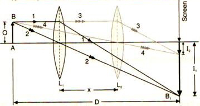If D is the distance between O and I and x is the distance between two positions of lens then focal length of the lens is given by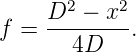The readers are advised to prove this result. Also, the method works only when D > 4f (prove it!).

Question. In the displacement method, a convex lens is placed in between an object and a screen if the magnifications in the two positions are m1 and m2 and the displacement of the lens between the two positions is x, then the focal length of the lens is?

#### 6.3 The Minimum Distance Method

This method is based on the fact that there is a minimum separation for object and image for a given lens. This can be shown if v - u is plotted against either u or v. A minimum is formed and this can be shown to occur at the point where u = -2f and v -u = 4f, that is, the minimum separation for object and image is 4f.

### References

   Focal length of a concave mirror by u-v method. https://goo.gl/PqDHRp. Good webpage at www.learncbse.in.

   Focal length of a convex lens by u-v method. https://goo.gl/PT39i1. Good webpage at www.learncbse.in.

   Focal length of concave mirror by u-v method. https://goo.gl/RqsX3D. Amrita Olabs Webpage.

   Focal length of concave mirror by u-v method. https://youtu.be/j8N1Z6338UQ. Amrita OLabs YouTube Video.

   Focal length of the concave mirror. https://youtu.be/5DbdWFAs8EI. Good YouTube Video from Edunovas.

   Focal length of the convex lens. https://youtu.be/f0bi0yl7uZU. Good YouTube Video from Edunovas.

   The measurement of the focal length of a lens. https://goo.gl/Rxy3gr. This webpage gives various methods to find focal length of a lens.

   NCERT book to find the focal length of a convex lens. http://ncert.nic.in/ncerts/l/lelm305.pdf. Download PDF from here.

   HC Verma. Concepts of Physics, volume Part 1. Bharati Bhawan, 1992.